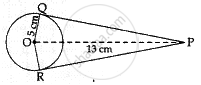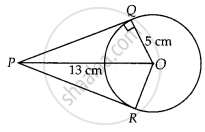# From a point P which is at a distance of 13 cm from the point O of a circle of radius 5 cm, the pair of tangents PQ and PR to the circle are drawn. Then the area of the quadrilateral PQOR is ______ - Mathematics

MCQ
Fill in the Blanks

From a point P which is at a distance of 13 cm from the point O of a circle of radius 5 cm, the pair of tangents PQ and PR to the circle are drawn. Then the area of the quadrilateral PQOR is ______

• 60 cm2

• 65 cm2

• 30 cm2

• 32.5 cm2

#### Solution 1

From a point P which is at a distance of 13 cm from the point O of a circle of radius 5 cm, the pair of tangents PQ and PR to the circle are drawn. Then the area of the quadrilateral PQOR is 60 cm2.

Explanation:OP2 = OQ2 + PQ2

169 = 25 + PQ2

PQ2 = 144

PQ = 12

Area PQOR = ar(AOPQ) + ar(AOPR)
= 1/2 × 12 × 5 + 1/2 × 12 × 5 = 60 cm2

#### Solution 2

From a point P which is at a distance of 13 cm from the point O of a circle of radius 5 cm, the pair of tangents PQ and PR to the circle are drawn. Then the area of the quadrilateral PQOR is 60 cm2.

Explanation:

Firstly, draw a circle of radius 5 cm with centre O. P is a point at a distance of 13 cm from O. A pair of tangents PQ and PR are drawn.∵ OQ ⊥ QP  .....[Since, QP is a tangent line]

In right angled ∆PQO,

OP2 = OQ2 + QP2

⇒ 132 = 52 + QP2

⇒ QP2 = 169 – 25 = 144

⇒ QP = 12 cm

Now, area of ∆OQP = /2 xx QP xx QO

= 1/2 xx 12 xx 5 = 30 cm2

∴ Area of quadrilateral PQOR = 2 × ar ∆OQP

= 2 × 30

= 60 cm2

Concept: Concept of Circle - Centre, Radius, Diameter, Arc, Sector, Chord, Segment, Semicircle, Circumference, Interior and Exterior, Concentric Circles
Is there an error in this question or solution?

#### APPEARS IN

NCERT Mathematics Exemplar Class 10
Chapter 9 Circles
Exercise 9.1 | Q 4 | Page 103
Share